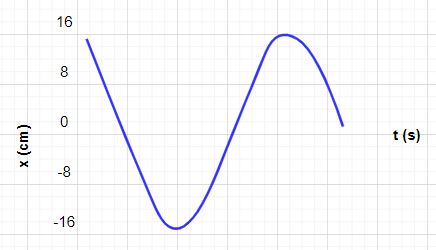# The figure gives the position as function of time, of 20 g block in simple harmonic oscillation...

## Question:

The figure gives the position as function of time, of 20 g block in simple harmonic oscillation on the end of a spring. The period of oscillations is 2.0 s. a. What is the amplitude of the oscillations?

b. What is the maximum speed of the oscillations?

c. What is the spring constant?## Spring:

Spring is defined as a mechanical component that is used to store energy in the form of elastic potential energy. Different types of springs are used in a machine like helical spring, torsion spring, leaf spring, etc. The strength of the spring depends on the spring index and spring stiffness.

Given Data

• The mass of a block is; {eq}m = 20\;{\rm{g}} {/eq}
• The time period of oscillation is; {eq}T = 2.0\;{\rm{s}} {/eq}

(b)

From the given figure, it is clearly observed that the amplitude is {eq}A = 16\;{\rm{cm}} {/eq} that indicates the maximum displacement of the block.

Find the angular speed of the block.

{eq}\begin{align*} \omega & = \dfrac{{2\pi }}{T}\\ & = \dfrac{{2\pi }}{2\; \rm s}\\ & = {\rm{3}}{\rm{.14}}\;{\rm{rad/s}} \end{align*} {/eq}

The formula of maximum speed of oscillation of the block is,

{eq}{V_{\max }} = \omega A {/eq}

Substitute the given value in the above equation to find the maximum speed of the oscillation.

{eq}\begin{align*} {V_{\max }} &= \omega A\\ & = 3.14\;{\rm{rad/s}} \times 16\;{\rm{cm}}\left( {\dfrac{{{\rm{1}}\;{\rm{m}}}}{{{\rm{100}}\;{\rm{cm}}}}} \right)\\ & = 0.5024\;{\rm{m/s}} \end{align*} {/eq}

So, the maximum speed of the oscillation is {eq}0.5024\;{\rm{m/s}} {/eq}.

(c)

The formula of the spring constant is,

{eq}k = {\omega ^2}m {/eq}

Here, {eq}k {/eq} is the spring constant and {eq}m {/eq} is the mass of an object.

Substitute the given value in the above equation to find the spring constant.

{eq}\begin{align*} k& = {\omega ^2}m\\ &= {\left( {{\rm{3}}{\rm{.14}}\;{\rm{rad/s}}} \right)^2} \times 20\;{\rm{g}}\left( {\dfrac{{{\rm{1}}\;{\rm{kg}}}}{{{\rm{1000}}\;{\rm{g}}}}} \right)\\ &= 0.197192\;{\rm{N/m}} \end{align*} {/eq}

So, the spring constant is {eq}0.197192\;{\rm{N/m}} {/eq}.Practice Applying Spring Constant Formulas

from

Chapter 17 / Lesson 11
3.4K

In this lesson, you'll have the chance to practice using the spring constant formula. The lesson includes four problems of medium difficulty involving a variety of real-life applications.